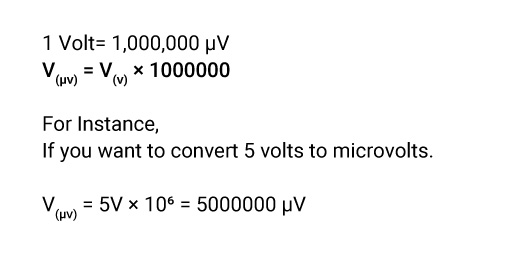## About Our V to µV Converter

"If you go more and deeper into things, you will eventually discover math there."

Gone are the days when you had to spend too much time on conversion and calculation. Now, you have a Handy converter to switch the volts to microvolts within a split second.

### What is the Meaning of Volts?

In simple words, a volt is a unit that measures electric force. It is the SI-derived unit for Voltage, i.e., the difference in Electrical potential energy/ Electromotive Force, per unit of charge, between two points of conductors.

One Volt equals the potential difference generated by moving one ampere of current against one ohm of resistance.

### What is the Symbol of Volts?

Volts can be represented by the Symbol V. For instance, you can write 1 volt as 1 V.

### How Can You Convert Volts to Microvolts?

Multiply the Voltage by the conversion ratio to convert volts to microvolts. The Voltage in microvolts is equal to the volts multiplied by 1,000,000. 1 Volt is equal to 1,000,000 microvolts.

### The Volts to Microvolts Conversion Formula.

You can use the below formula for Volts to Microvolts Conversion,The best thing is volts to microvolts conversion has become easy with the help of volts to microvolts converter.

### How to Use Our Volts to microvolts Converter?

Now, you can convert V to µV using our tool in the blink of an eye.

• Step1: Click on our website.
• Step2: Now enter the value in volts to be converted into microvolts.
• Step 3: Click on "Convert," and there you go.

### How Many Volts Are in a Microvolt?

1 Microvolt = 1/1,000,000 Volt.

1 Microvolt = 10-6 Volts.

One microvolt is equal to 1/1,000,000 of a volt, which is the potential difference generated by moving one ampere of current against one ohm of resistance.

### What is the Difference Between Volt & Microvolt?

Volt is the ST unit of Voltage (electrical potential difference) and electromotive force. 1 volt= 1,000,000 Microvolts. Volt is denoted by V.

Microvolt is also an SI derived unit for Voltage, but it is the multiple of Volt. Microvolt is the 1/1,000,000 part of a volt and is denoted by µV.

### What is the Volt Unit of Measure?

Volt (V or E) is the measuring unit of Voltage (electrical potential difference) and electromotive force.

### Examples of Converting Volts to Microvolts.

V(µV) = 1 V 10 6= 1,000,000 µV

V(µV) = 3 V 10 6 = 3,000,000 µV

V(µV) = 8 V 10 6 = 8,000,000 µV

### Volt to Microvolt Conversion Table.

Volts Microvolts
0.0000001V 0.1 µV
0.000001V 1 µV
0.00001V 10 µV
0.0001V 100 µV
0.001V 1,000 µV
0.01V 10,000 µV
0.1V 100,000 µV
1V 1,000,000 µV